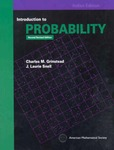## Open Textbooks#### Title

Introduction to Probability

#### Contributors

Charles M. Grinstead - Author / J. Laurie Snell - Author

#### Description

Probability theory began in seventeenth century France when the two great French mathematicians/ Blaise Pascal and Pierre de Fermat/ corresponded over two problems from games of chance. Problems like those Pascal and Fermat solved continuedto influence such early researchers as Huygens/ Bernoulli/ and DeMoivre in establishing a mathematical theory of probability. Today/ probability theory is a wellestablished branch of mathematics that finds applications in every area of scholarlyactivity from music to physics/ and in daily experience from weather prediction topredicting the risks of new medical treatments. This text is designed for an introductory probability course taken by sophomores/juniors/ and seniors in mathematics/ the physical and social sciences/ engineering/and computer science. It presents a thorough treatment of probability ideas andtechniques necessary for a form understanding of the subject. The text can be usedin a variety of course lengths/ levels/ and areas of emphasis. For use in a standard one-term course/ in which both discrete and continuousprobability is covered/ students should have taken as a prerequisite two terms ofcalculus/ including an introduction to multiple integrals. In order to cover Chapter 11/ which contains material on Markov chains/ some knowledge of matrix theoryis necessary. The text can also be used in a discrete probability course. The material has beenorganized in such a way that the discrete and continuous probability discussions arepresented in a separate/ but parallel/ manner. This organization dispels an overlyrigorous or formal view of probability and o?ers some strong pedagogical valuein that the discrete discussions can sometimes serve to motivate the more abstractcontinuous probability discussions. For use in a discrete probability course/ studentsshould have taken one term of calculus as a prerequisite. Very little computing background is assumed or necessary in order to obtain fullbenefits from the use of the computing material and examples in the text. All ofthe programs that are used in the text have been written in each of the languagesTrueBASIC/ Maple/ and Mathematica.

#### Subject 1

Mathematics - Applied

Engineering

#### Publisher

American Mathematical Society

#### Resources

Open Textbook Library